# Introduction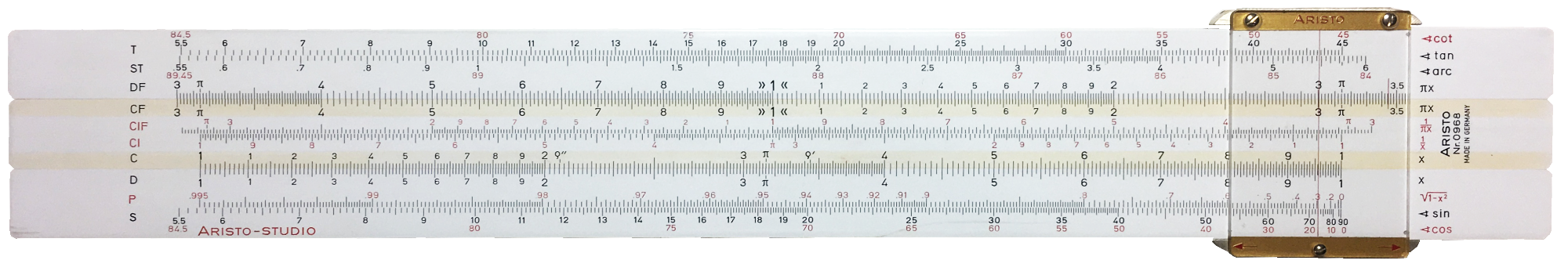Front view of a “Studio” model slide rule by Aristo

Slide rules were heavily used in engineering before the appearance of cheap hand-held electronic calculators.  They allowed many fairly precise calculations to be made in reasonable time.  Engineering work involves lots of computations, and there there were only two alternatives:  using mechanical calculators if precision was important, or using pre-computed tables if the use of trigonometric functions and such was needed.

For much engineering work it is not necessary to get results to a high degree of precision, simply because the data one starts out from are not very well known in the first place.  A length can be measured to a fair precision, but not the strength of a given wooden beam, at least not without destroying it.  Engineers compensate for this variability by using safety margins.

Nevertheless, the design of a bridge, a building, an internal combustion engine or an electric motor does imply large numbers of computations, mostly involving multiplications, divisions, square roots, trigonometric functions and the like.  While it is not necessary to get results with many digits of precision, the speed and ease to get a sufficiently good value are important.

Slide rules were the solution and they are surprisingly accurate.

(there is a working version in the examples section)

# Numeric calculations

Before we start on slide rules we must observe some arithmetic effects.

## Look at these calculations:

• 12×34=408
• 120×340=40800
• 1.2×34=40.8
• 1.2×3.4=4.08
• 0.012×34=0.408

There are two important observations:

1. the values are very different, but the digits are all the same
2. no number starts with a zero except to indicate that it is smaller than 1:  the value 0034 is identical to 034 and to 34, we never use leading zeros unless there is a decimal point.

## You cannot multiply

There is no device, no method, that arrives at the result of a multiplication except by splitting it up into additions.

This is true for classic hand-written multiplications, but also for computers.  You may argue that the result of 5×7 is not arrived at by adding 7 to itself 5 times, but that is because you learned by heart that 5×7=35.  For small numbers you do not multiply, you look up the result in a “table” that you stored in your memory, learned by heart in primary school.  For larger numbers you use this table plus some additions.

## But you can add

Consider this table:

• 2=2
• 2×2=4
• 2×2×2=8
• 2×2×2×2=16
• 2×2×2×2×2=32
• 2×2×2×2×2×2=64
• 2×2×2×2×2×2×2=128
• 2×2×2×2×2×2×2×2=256

We can write this more concisely by just giving the number of times we multiply by 2 by itself, indicating that number at the top right of each number 2.  That little number is called the exponent:

• 21=2
• 22=4
• 23=8
• 24=16
• 25=32
• 26=64
• 27=128
• 28=256

So 26 means “multiply 2 by itself 6 times”.  The results are called powers26 is pronounced 2 to the power 6 (or “two to the sixth power” or simply “2 to the sixth”)

Now let’s multiply 8 by 16:

8×16 = 2×2×2  ×  2×2×2×2 = 23 × 24 = 27 = 23+4

Rather unsurprisingly, the number of times we have to multiply 2 by itself to get the result is the sum of the number of times we needed for the factors.

To find the exponent of the result of the multiplication, we add the exponents of the factors!

What is 4×8?  Easy:  from the table we see that the exponents are 2 and 3 respectively, and 2+3=5 so we look for the number with exponent 532.

We transformed a multiplication into an addition, which is much easier to do.

## Division by subtraction

Say we divide 128 by 16:

$128 16 = 27 24 = 2×2×2×2×2×2×2 2×2×2×2 = 27−4 = 23 = 8$

It is sufficient to subtract the exponents, because of the cancelling of the factors 2 in the numerator and the denominator.

Important note:  because of this, we can, and should, write:

$2 2 = 21 21 = 21-1 = 20 = 1$

Therefore our previous list can be extended by a first line giving the “zeroth power of 2”:

• 20=1
• 21=2
• 22=4
• 23=8

This is true for any number:  its zeroth power is 1.

## Logarithms

Using addition instead of multiplication worked fine for this table of powers of 2, and you are also familiar with powers of ten:  you know that 1'000 × 100'000 = 100'000'000 because you know you can add the zeros:

1'000 = 103, 100'000 = 105, 100'000'000 = 103+5 = 108

But what about multiplying 12 by 3416 can be expressed as a power of 2 and 1'000 as a power of 10, but what about 12 and 34?

If we observe that 10=101 and 100=102 then maybe 34=10x where x is some number larger than 1 but smaller than 2?

Without going into further details, yes indeed, 34=101.531479….  Fractional powers are difficult to compute, but they exist.  We have:

12=101.079181…, 34=101.531479… but note that the exact fractional exponent has an infinite number of decimals, in practice we have to stop after a few digits.

Now, just like for the examples with whole powers, we should have that:

101.079181×101.531479 = 101.079181+1.531479 = 102.61066 = 407.999847

The correct result of 12×34 is of course 408, but using the addition method on not totally exact exponents gave a result that is off by less than 1 in a million.  Largely good enough:  as good as measuring the width of your house to be ten metres plus or minus a hair’s breadth!

The fractional powers are called logarithms and they are the basis of how slide rules work.

# Adding with rulers

We can use two rulers bearing identical numbered marks at regular intervals, to add numbers by sliding the rulers next to each other:

In the drawing the top ruler is displaced from the right of the bottom ruler, in such a way that its 0 mark comes opposite the 2 mark of the bottom ruler.  It is then clear that under the 3 mark of the top ruler lies the 5 mark of the bottom ruler:  the distance 2 plus the distance 3 is equal to the distance 5, which we can read on the bottom ruler:  2+3=5.

But likewise we can subtract:

With the 3 above the 5, we remove 3 from 5 and get to 2, which we can read on the bottom ruler:  5−3=2.

## Multiplying

Instead of using successive integers, let’s label the marks with the powers of 2 of our first example of multiplication by addition, and let us not forget that we should start with the zeroth power, which is 1:

Now we have something useful:  we can multiply powers of 2.  4×2=8, 4×16=64 and so on.  We see these results by sliding the top ruler so that its 1 is over the value of the first factor (4 in the figure above), then read the value on the bottom ruler that is under the second factor, e.g. 16, to obtain the result 64.

It is from this sliding of two rulers that the name slide rule is derived.

Slide the top more to the right to see multiplication by 8:

Since the top ruler now has its 1 over the 8 of the bottom ruler, we are now multiplying by 8: 2×8=16, 4×8=64, …

Likewise, we can divide:  128/16=8 since putting the 16 above the 128 and then going to the left until the 1 shows us 8.

## Other numbers

By some guesswork, we could put the number 3 somewhere between the 2 and 4, and we could put 5, 6 and 7 somewhere between the 4 and the 8, and so on (as we advance, we have to cram more and more numbers in between the already existing ones).

If we placed the numbers 1 to 10 correctly by using their logarithms, we get what is called a logarithmic scale:

Note that the distance 1—2 is the same as the distance 2—4 and the distance 4—8, and this is so because it’s nothing other than the regular distances between powers of the same number.  The distance 1—3 is therefore also the same as the distance 3—9 because 9=3×3=32.

It is a bit squashed up, more pleasing:

And now multiply:  slide the top ruler’s 1 over the bottom’s 3, observe that 2×3=6, 3×3=9.  We need more marker lines, e.g. if we had a line for 2.5 we would see it sit above the line for 7.5 (2.5×3=7.5)

# Forgetting magnitude

The above ruler only goes from 1 to 10.  How far do we want to go?  Consider:

This does not make much sense:  the stretch from 10 to 100 looks the same as that from 1 to 10, or 100 to 1000 etc. and everything is squashed up making it difficult to get good precision unless we make the ruler so long that it becomes unwieldy.

The magnitude of the result less important than the precision, i.e. the number of digits.

We only need the numbers from 1 to 10, remembering that further to the right (or to the left) the marks will be in the same positions with the digits repeated, except that the decimal point moves.  Thus we stick to a single, large scale, with as many intermediate markers as possible for precision.  After adding the logarithmic positions for the numbers 1.1, 1.2, 1.3, … we get:

The imagined repetition of this scale to its left and right is also the reason why the label of the last mark of the above scale is not 10, but simply 1 (though some rules do use 10).  Omitting the zero reminds us that we need no more scales. Again, we do this because we are mainly interested in the digits, not the magnitude of the number.  Indeed, even in the rulers for multiplying powers of 2, it did not matter whether we were calculating 4×8 or 40×8, in both cases the digits of the result would have started with a 3 followed by a 2. We will have to keep this in mind, because it is the downside of calculating with a slide rule:  we get the digits, but not where the decimal point sits.

Obviously we need even more intermediate markings if we want to get more digits precision.  This is a picture of a logarithmic scale of a real slide rule:

And a close-up: (scroll horizontally)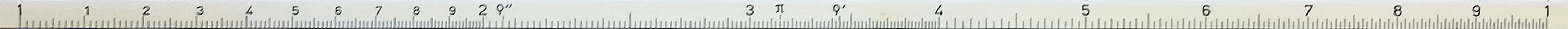a real scale from a 30cm slide rule

Unless you have a very wide screen, the close-up probably shows only the markings from 1.0 to less than 2.0.  Effectively there are ten intermediate numbers engraved between the 1 and the 2, they give the positions of 1.1, 1.2, 1.3 and so on.  Between each of those are another ten marks, for 1.01, 1.02 etc.

Looking at the section between 9 and 10: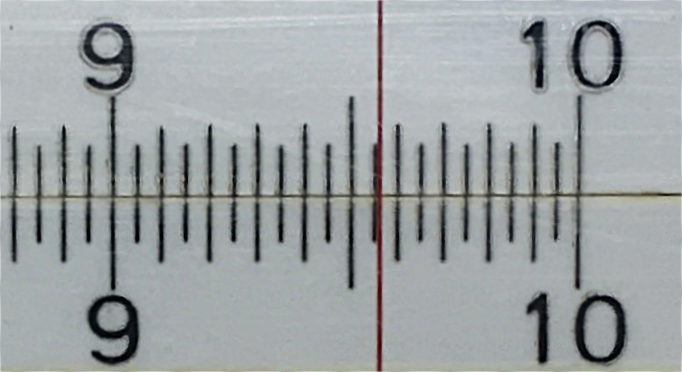9 to 1

With some practice one can easily find the position of, say, 956 (marked with the cursor’s thin red line) and that means a good approximation to 3 digits is possible with a 25cm slide rule (but see also clever trick below).

The important thing to note is that the red line marks the position of 456, but also 4.56, 456000, 0.0456 and indeed any number whose first three digits are 4, 5 and 6.

# Examples

Take again the multiplication 12×34.

On the slide rule in the image below, there are many scales, each labelled on the left.  We will only use the D and C scales (yellow circles) as they are normal logarithmic scales.  The red arrow on the D scale indicates the position 12.  The slide which carries the C scale has been moved to the right so that its first 1 is exactly above the 12.

Slide rules usually have a cursor with a thin vertical line, to aid in accessing scales other than C and D.  Here the cursor has been moved to the position indicated by the blue arrows.  The top arrow is at position 34 on the C scale, the fine line on the cursor is at that same position.  The bottom blue arrow indicates something more than 405 but less than 410, it looks like 408.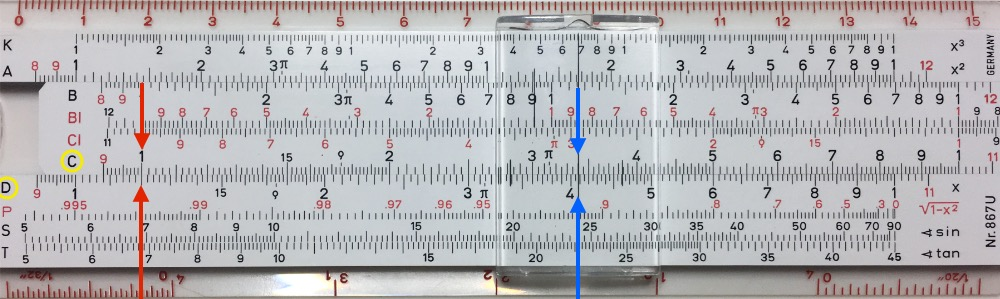12×34=408 or 408/34=12

Division is equally simple:  to divide 408 by 34, put the 34 above the 408, then look under the 1 to find 12.  That’s because we now used the rules to subtract the logarithmic distances.

## A working version

Below are just the C and D scales of a 25cm slide rule.  It helps to have a large screen so you can make the browser window wide enough.

You can drag the C scale left and right, and for precise adjustment you can click:  a normal mouse click will move the C scale by 1 unit right, and a shift-click will move it left by one unit.  The scale itself is 2000 units wide.  (don’t click too fast or you will cause a double-click)

# Other Scales

Apart from the C and D scales, which are used for multiplication and division, there were many other useful scales.  We discuss folded scales below.  Most slide rules carried scales for squares (and therefore square root if used in the other direction), sine, tangent, exponential, $\sqrt{1-{x}^{2}}$, cube, and many specialised engineering functions.

Classic lengths of the logarithmic scale on slide rules are 12.5cm (pocket), 25cm (normal) 50cm (full).  The body was of course somewhat longer than the scales.  Slide rules came with different sets of scales, hence were given names like “Studio”, “Junior”, “Darmstadt” and so on.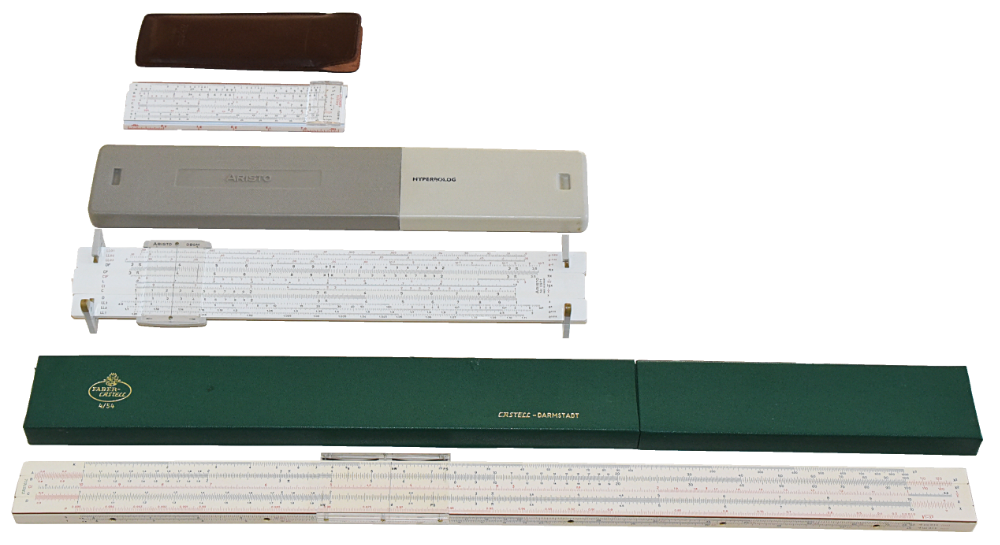a 12.5cm pocket Aristo Darmstadt, a 25cm Aristo Hyperbolog on its feet and a 50cm Faber-Castell Darmstadt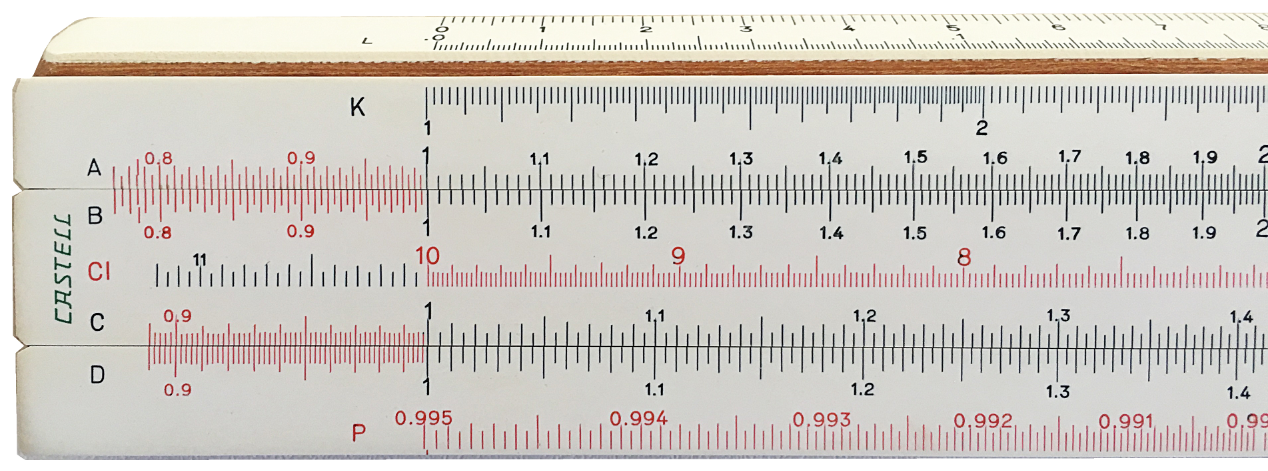engravings of the Faber-Castell

Because the material must not warp with time or deform with temperature and humidity, early versions were made of ivory, later ivory glued to pear wood or bamboo, then celluloid plastic on pear wood (like the Faber-Castell in the photo) or bamboo (Japan) and still later from high-quality plastics.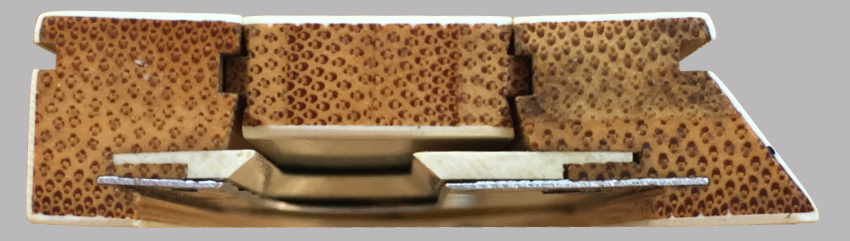end view of Japanese bamboo based slide rule

The friction with which the centre ruler slides in the body is also very important:  it must not be loose, but it must not be so tight that it causes stick-slip, and this friction should not change over the lifetime of the rule.  Slide rules, when properly cared for, last a lifetime.  The five slide rules of which there are images in this page are all mine and one of them I have very heavily used from 1964 to 1971.  You can find used slide rules fairly easily, although they will get rarer.

# Problems and Solutions

## The Last Digit

In the previous example of finding 12×34 we were not quite sure about the last digit.  However, we know from our memorised multiplication tables that the last digit of 12×34 will necessarily be the multiplication of their last digits 2×4=8.  Therefore the result is indeed 408.  One can also see very quickly if the result is going to be odd or even.

## The Magnitude

However, while the slide rule tells us the digits of the result are 4,0,8 it does not tell us whether it is 408 or 40.8 or 4080.  But we can approximate 12×34 to, say, 10×36 by rounding the 12 down to 10 and making the 34 slightly larger to 36.  The result of 12×34 can therefore not be too far away from 10×36=360, so the result must be 408 and not 40.8 nor 4080.

## The “Other-One”

Say we want 21.5×7.3

Naively we would start by positioning the slide thus (first 1 of scale C over 21.5 of scale D):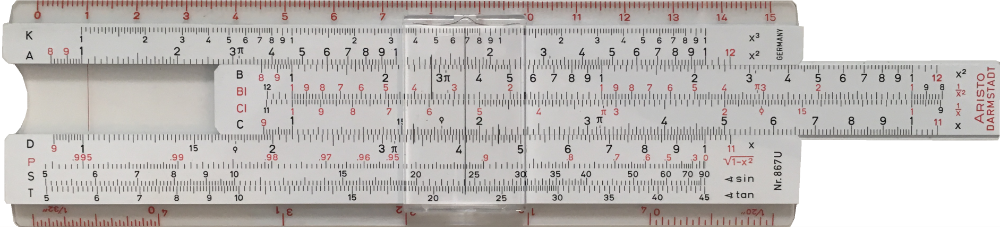21.5×73

But the position of 73 on the C scale now lies off the right of the D scale.

If under the C scale there were another scale extending the D scale, then we could do it.

On that scale the position under the last 1 of the C scale would of course be 21.5:21.5×73 with extended scale

Therefore, it’s the same as if we slid the ruler to the left, to position the C scale’s other 1 over the 21.5: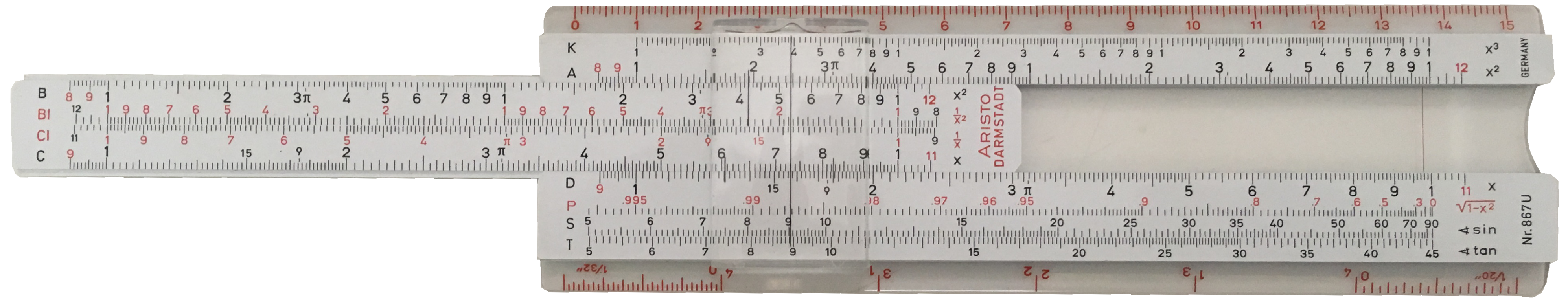21.5×73 with “other one”

And then we read digits 157, whereby we know that for magnitude 20×70=1400 so it must be 1570 and since 3×0.5=1.5, the last digit must be 0.5.  So the precise result must be either 1569.5 or 1570.5.  It is in fact 1569.5, but 1570 is good enough:  it is off by only 5 in 15000 or less than 0.04%.

## Halves and other fractions

The previous example used a pocket slide rule with 12.5cm scales.  Using a larger slide rule we might perhaps get a better result, but the next size up has another interesting problem:  there is no mark at 21.5, only at 21.4 and 21.6: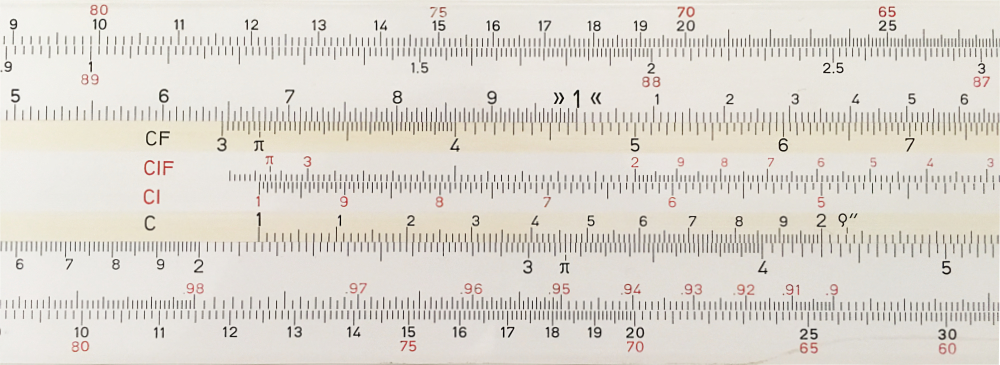21.5 not marked

We must try by sight to position the 1 between the 214 and the 216 marks.  But 21.5×2=43 so we may position the C scale more precisely by putting its 2 above 43 rather than trying to put its 1 between 21.4 and 21.6.

This is rather splitting hairs, and I have never used this positioning trick in real work.

## Shifted or “Folded” Scales

Look again at the example where we needed to shift the slide to the left in order to get something under the 73.

Some slide rules have “folded” or shifted C and D scales.  They are labelled CF and DF and sit at the other side of the slider: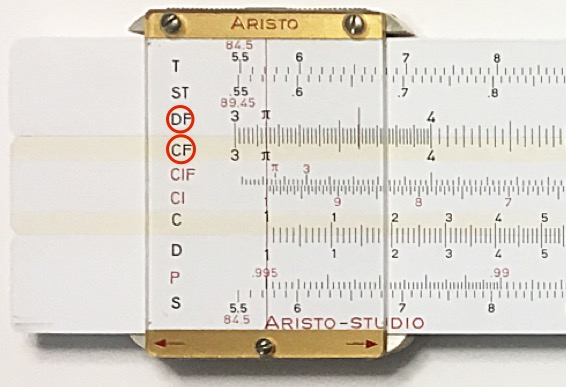Shifted scales CF, DF

They are just the same as the C and D scales, but shifted by π=3.14159.  As you can see, if the cursor is over the first 1 of the D scale, it indicates π on the DF scale.

Sliding will let you multiply with the CF and DF scales just the same way as with the C and D scales.

These extra scales are however useful for two reasons.

### Multiplying by π

It needs no explanation that the first use is to multiply by π without having to shift anything at all.

### Avoiding the “other-one” problem.

It so happens that π is also close to $\sqrt{10}$ and that lies halfway along the C scale.

It may need some thinking, but therefore the CF and DF scales can be used to avoid the problem where the desired second factor lies off the scale:  if the first factor is on the first half of the D scale, (i.e. between 1 and 3), use the first 1 of the C scale to position the slider;  if it is on the second half, i.e. between 3 and 10, use the second 1 of the C scale.  Using 3 is good enough because the shifted scales extend a little on both sides.  Now you will always find the second factor within reach, either on the C or on the CF scale:73 is still usable on the CF scale

And the result of 21.5×7.3 can be read on the DF scale as 1570 (but even on this larger slide rule, the mark is not significantly to the left).  So we can now position the slider without consideration for the second factor, as long as we never slide it more to the left or right than π.

There is another way to avoid the “other-one” problem:  use a circle.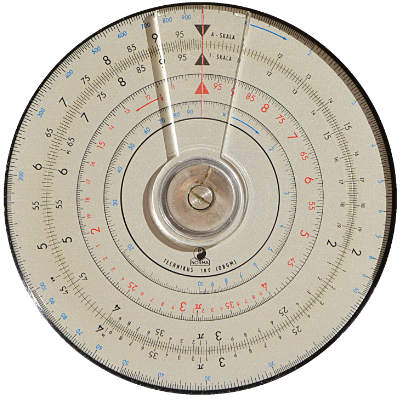a circular slide “rule”

Circular rules avoid the “other-one” problem but they are more difficult to slide, must often be rotated to be read, are more awkward to carry around and have less precision on scales with smaller diameters.  There are also cylindrical versions, see the links to the museums below.

# Proportionalities

One useful application of the slide rule is to compute proportionalities.  This solves the classic “rule of three” very fast.

The “rule of three” crops up in many everyday problems like this one:  if 8 apples cost 5€, how much will 20 apples cost?

The first step, or rule, is to say:  8 apples cost 5€

The second step is to say that therefore 1 apple costs 5/8€ or 0.625€ (divide to get to a single apple).

The third and final step is then to say that if we know the cost of one, then the cost of 20 is 20×0.625=12.5€ (multiply by the number of apples)

With a slide rule we get the division and multiplication at the same time.  Place the 8 above the 5: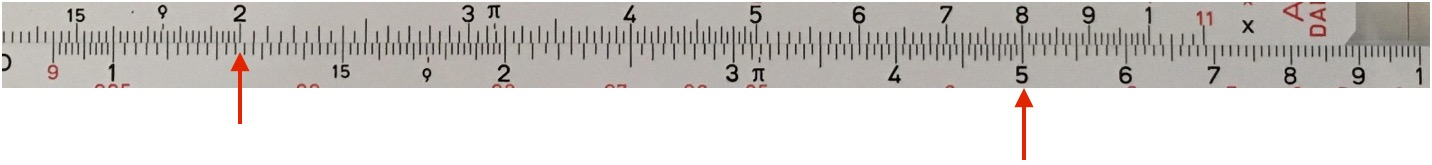all 8/5 proportions

That indicates 8 apples cost 5€.  You can now see that 1 apple costs 0.625 (under the second 1 of the C scale, to the right of its 8) but also that 4 apples cost 2.5€, and 20 apples cost 12.5€ and whatever else you would like, including fractional apples.  All 8/5 proportions are simultaneously available!

Proportionality can be helpful when making a scale drawing (or, at least, that used to be helpful before digital drawing programs which can do scale reduction automatically).

# Other Sites

You may want to look at: